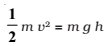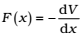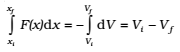Potential energy is the ‘stored energy’ by virtue of the position or configuration of a body. The body left to itself releases this stored energy in the form of kinetic energy.

Let us make our notion of potential energy more concrete.

The gravitational force on a ball of mass m is mg . g may be treated as a constant near the earth surface. By ‘near’ we imply that the height h of the ball above the earth’s surface is very small compared to the earth’s radius RE(h <<RE) so that we can ignore the variation of g near the earth’s surface

Let us raise the ball up to a height h. The work done by the external agency against the gravitational force is mgh. This work gets stored as potential energy. Gravitational potential energy of an object, as a function of the height h, is denoted by V(h) and it is the negative of work done by the gravitational force in raising the object to that height.

V (h) = mgh

If h is taken as a variable, it is easily seen that the gravitational force F equals the negative of the derivative of V(h) with respect to h. Thus,The negative sign indicates that the gravitational force is downward. When released, the ball comes down with an increasing speed. Just before it hits the ground, its speed is given by the kinematic relation,

v2 = 2gh

The equation can be written aswhich shows that the gravitational potential energy of the object at height h, when the object is released, manifests itself as kinetic energy of the object on reaching the ground.

Physically, the notion of potential energy is applicable only to the class of forces where work done against the force gets ‘stored up’ as energy.

Mathematically, (for simplicity, in one dimension) the potential energy V(x) is defined if the force F(x) can be
written asThis implies thatThe work done by a conservative force such as gravity depends on the initial and final positions only.

The dimensions of potential energy are [M L2 T-2] and the unit is joule (J), the same as kinetic energy or work. To reiterate, the change in potential energy, for a conservative force, ΔV is equal to the negative of the work done by the force.